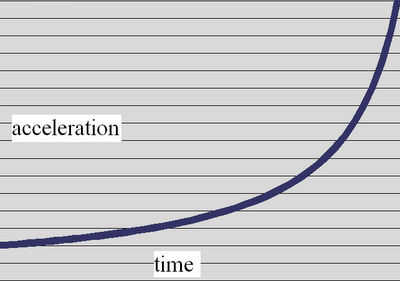## 2006-11-29

### Deriving The Rocket Equation

This is probably a job interview question somewhere. I was just thinking about it
and decided to try it out myself, with no regard to convention beyond what is already intuitive for me.

The question is, you have rocket with a certain amount of mass, some of which is fuel. The rocket consumes fuel at a constant rate in order to produce a constant thrust. If the rocket starts at time zero, how fast is it going and what is its displacement at some later time?

So the lets say the fuel consumption rate is a positive number k, in units of kg/sec.
The fueled mass of the rocket is Mo.
Therefore the mass at a later time t is
M(t) = Mo - kt

From physics it is known that F=MA. Our F is constant, we'll just call it F, its units are kg*m/s^2. Acceleration is the thing that will change, intuitively acceleration should increase as the mass goes down as fuel is consume.

A= F/M = F/M(t) = F/(Mo - kt)

Looking at that equation makes sense- the denominator gets smaller therefore A goes up as time increases.So the trick to finding velocity is integrating that curve and position is found by integrating again. Easy numerically, but of course we want a nice analytical answer.

I've forgotten a lot of the little tricks of calculus, which is just as well since I want to solve this as intuitively as possible anyway. The most I did lookup to remind myself was to find that the integral of 1/x is ln(x) and that ln(x/y) = ln(x) - ln(y), and that to have definite integral over a specified interval (time 0 to time t1 here) you can subtract the indefinite integral of the lower bound from the indefinite integral of the higher bound.

We don't have a 1/x equation, at first glance, we have a 1/(constant- x) equation- so how do you integrate that? The trick is to make our equation into the form of 1/x.

1/x backwards to our acceleration graph:

So we need to reverse our graph to make it look like that and then we'll have the integral. Instead integrating with respect to time, we'll integrate with respect to (Mo-kt) and do it from the lower bound Mo-ktf (tf is the final t) to Mo (Mo - k to, to is zero so it's just Mo).

So we end up with

Integral of F/(Mo-kt) d(Mo-kt) = F * Integral of 1/(Mo-kt) dkt
(since Mo does not change the interval d(Mo-kt), it drops out). Unfortunately the units of this integral is going to be kg*m/s^2 * 1/kg * kg/s * s (the units of the F times the units of the 1/ mass times the units of the k times the units of the t) = kg*m /s^2 - we want velocity not force! In order to cancel out the k in dkt inside the integral we divide F by k outside of the integral.

f/k units is m/s - a velocity which we should return to later.

f/k * ( ln(Mo) - ln (Mo - ktf) ) = f/k * ( ln (Mo/(Mo - ktf)) )

Or if we say Mf = Mo - ktf, then v(t) = f/k * ln(Mo/M(t)).

Exhaust Velocity

Going slight further in, we should look at what is happening in order to accelerate the rocket. Rockets operate by throwing mass out the back end at a high velocity- throwing out a little bit of mass a high velocity adds a little velocity to your rocket with a big mass- and there's an equation of momentum to show
how those four things are related:

m1 * deltaV1 = m2 * deltaV2

We know we're burning m1 = k amount of fuel every second, and that since we're not doing any nuclear reactions the k amount of fuel is still there in mass form- it's just moving out the back end at high velocity. We know the mass of the rocket Mo or M(t) and its acceleration (delta-v) for that moment is a(t). So we have three of the four quantities, the delta-v of the exhaust fuel is what we want. Since the exhaust velocity doesn' t change we can just use Mo = M(0) and a(0)*1 second = F/Mo*1second (to simplify we assume that acceleration doesn't change much over 1 second).

m2*deltaV2 / m1 = deltaV1
Mo*F/Mo* 1sec / ( k * 1sec ) = deltaV1
exhaust velocity deltaV1 = F/k - the same f/k in the above rocket equation. So instead of saying f/k we can say 'exhaust velocity'.